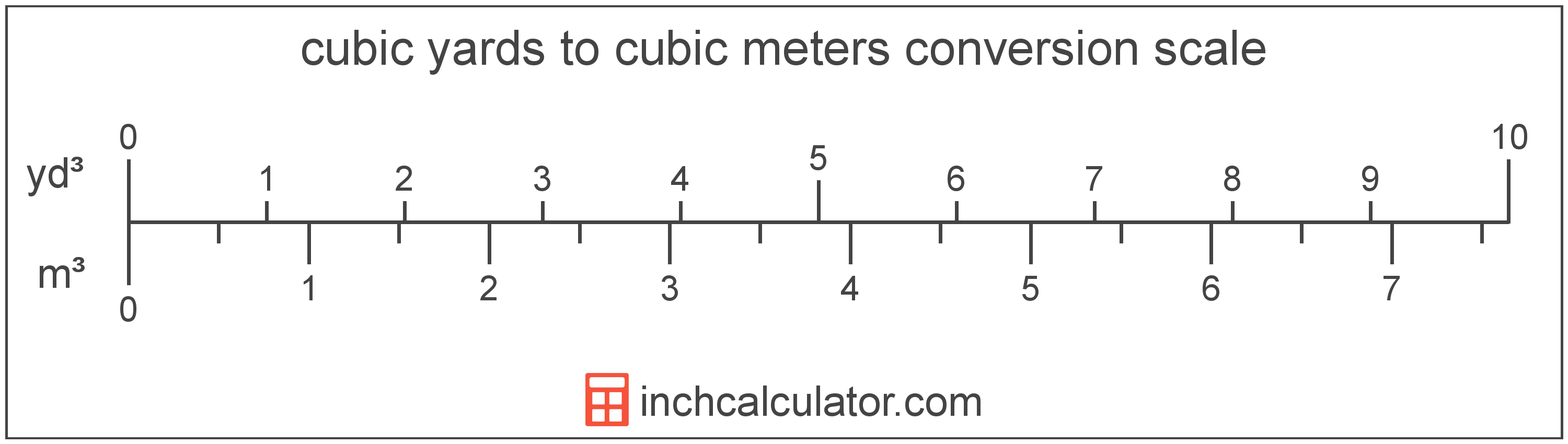# Cubic Yards to Cubic Meters Converter

Enter the volume in cubic yards below to get the value converted to cubic meters.

(find cubic yards)
Results in Cubic Meters:1 yd³ = 0.764555 m³

Do you want to convert cubic meters to cubic yards?

## How to Convert Cubic Yards to Cubic Meters

To convert a measurement in cubic yards to a measurement in cubic meters, multiply the volume by the following conversion ratio: 0.764555 cubic meters/cubic yard.

Since one cubic yard is equal to 0.764555 cubic meters, you can use this simple formula to convert:

cubic meters = cubic yards × 0.764555

The volume in cubic meters is equal to the volume in cubic yards multiplied by 0.764555.

For example, here's how to convert 5 cubic yards to cubic meters using the formula above.
cubic meters = (5 yd³ × 0.764555) = 3.822774 m³### How Many Cubic Meters Are in a Cubic Yard?

There are 0.764555 cubic meters in a cubic yard, which is why we use this value in the formula above.

1 yd³ = 0.764555 m³

## What Is a Cubic Yard?

The cubic yard is a unit of volume that is equal to the space consumed by a cube with each edge measuring one yard.

The cubic yard is a US customary and imperial unit of volume. A cubic yard is sometimes also referred to as a cubic yd. Cubic yards can be abbreviated as yd³, and are also sometimes abbreviated as cu yard, cu yd, or CY. For example, 1 cubic yard can be written as 1 yd³, 1 cu yard, 1 cu yd, or 1 CY.

You can calculate volume using a cubic yardage calculator if you have the dimensions of a space or object.

## What Is a Cubic Meter?

One cubic meter is equal to the volume of a cube with each edge measuring one meter.

The cubic meter, or cubic metre, is the SI derived unit for volume in the metric system. Cubic meters can be abbreviated as , and are also sometimes abbreviated as cu m, CBM, cbm, or MTQ. For example, 1 cubic meter can be written as 1 m³, 1 cu m, 1 CBM, 1 cbm, or 1 MTQ.

You can use our cubic meters calculator to calculate the volume of a space.

## Cubic Yard to Cubic Meter Conversion Table

Table showing various cubic yard measurements converted to cubic meters.
Cubic Yards Cubic Meters
1 yd³ 0.764555 m³
2 yd³ 1.5291 m³
3 yd³ 2.2937 m³
4 yd³ 3.0582 m³
5 yd³ 3.8228 m³
6 yd³ 4.5873 m³
7 yd³ 5.3519 m³
8 yd³ 6.1164 m³
9 yd³ 6.881 m³
10 yd³ 7.6455 m³
11 yd³ 8.4101 m³
12 yd³ 9.1747 m³
13 yd³ 9.9392 m³
14 yd³ 10.7 m³
15 yd³ 11.47 m³
16 yd³ 12.23 m³
17 yd³ 13 m³
18 yd³ 13.76 m³
19 yd³ 14.53 m³
20 yd³ 15.29 m³
21 yd³ 16.06 m³
22 yd³ 16.82 m³
23 yd³ 17.58 m³
24 yd³ 18.35 m³
25 yd³ 19.11 m³
26 yd³ 19.88 m³
27 yd³ 20.64 m³
28 yd³ 21.41 m³
29 yd³ 22.17 m³
30 yd³ 22.94 m³
31 yd³ 23.7 m³
32 yd³ 24.47 m³
33 yd³ 25.23 m³
34 yd³ 25.99 m³
35 yd³ 26.76 m³
36 yd³ 27.52 m³
37 yd³ 28.29 m³
38 yd³ 29.05 m³
39 yd³ 29.82 m³
40 yd³ 30.58 m³